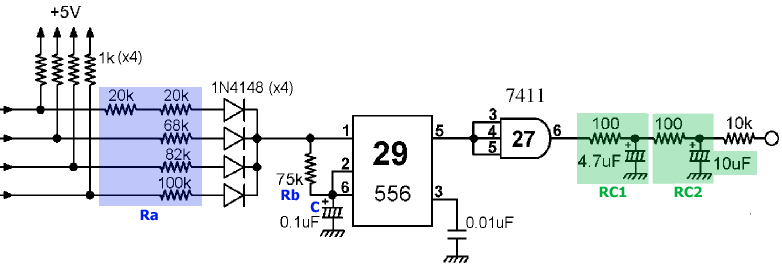# Step

The "walking" sound of the invaders. There are four inputs to the circuit, each connected to a different resistor, and the game turns them on sequentially one at a time to produce four different tones.The most important component here is the 556 timer, which is actually two 555 timers packaged into one. Here it is equivalent to a single 555 timer (the other one is used for the extended play sound) used in astable mode. In this typical configuration, the timer is enabled by pin 1 going active and produces a square wave at pin 5.

The parameters of the output wave are controlled by the value of resistors Ra and Rb (in ohms) and the capacitor C (in farads):

t0 = log(3) (Ra+Rb) C = 1.1 (Ra+Rb) C
t1 = log(2) (Rb) C = 0.693 (Rb) C
t2 = log(2) (Ra+Rb) C = 0.693 (Ra+Rb) C

Initially the wave stays high for t0 seconds, then it goes low for t1 seconds, high again for t2 seconds and so on alternating between t1 and t2. (For an explanation of the formulas, including why the very first period is longer than the others, see the 555 datasheet).

Before going into the amplifier, the square wave passes thru two RC filters, which "soften" its edges a little. There are tons of material around on these filters, so here I will only point out that it is not difficult to implement them in digital form. In fact if we have a sequence of samples x(1)...x(n) then the output of the filter at the n-th sample is:

y(n) = a x(n) + b y(n-1)

where a and b derive directly from the RC values of the filter:

b = exp(-1 / (R C SamplingRate))

a = 1 - b

Note that we use the current sample and the sample previously output by the filter itself.

So, the circuit can be emulated by computing the periods of the square wave with the formulas above (where Ra is variable and selected at runtime), generating the signal and then filtering it thru the two RC filters RC1 and RC2. Not bad, considering that this circuit alone plays four sound effects (out of a total of ten).